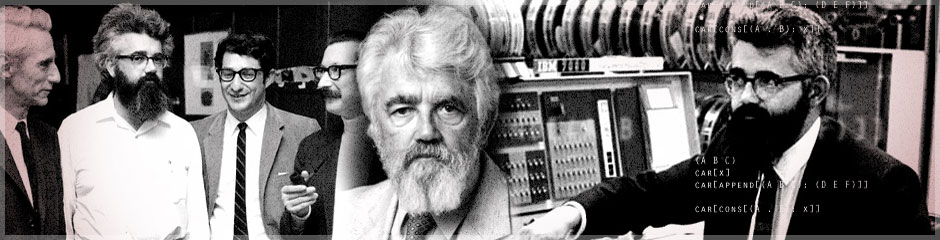Professor John McCarthy
Father of AI# Tough Nut for Proof Procedures

Here's the article which was a 1964 Stanford AI Memo. After the original memo, several people offered different proofs of the theorem including Shmuel Winograd, Marvin Minsky and Dimitri Stefanyuk - none published, to my knowledge. Winograd claimed that his proof was non-creative, because it didn't use an extraneous idea like the colors of the squares. This set off a contest to see who could produce the most non-creative proof.

Minsky's idea was to start with the diagonal next to an excluded corner ssquare, note that 2 dominoes had to project from it to the diagonal with three squares, and from there 1 domino to the four square diagonal, etc. Coming from the other end also leaves only six of the eight squares in the long diagonal covered. Minsky's proof gets high points for non-creativity, because it is specific to the 8 by 8 board. (Using the colors it is easy to show that a Minsky style proof will work for any even sized board.)

It recently (1995) occurred to me that Minsky's proof could be made into a propositional calculus proof with a succession of lemmas, because each statement about the number of dominoes projecting from a diagonal was a disjunction of feasible size of a few of the 256 propositional variables asserting that a dommino projected in a given direction froom a given square.

I therefore gave the problem to Mark Stickel at SRI and also to Dan Pehoushek, a former graduate student in our department who had propositional calculus provers. Neither bothered with the lemmas but gave the prover the sentence in 256 variables. Each prover did the problem. I forget whether Stickel said his prover took ten seconds or ten minutes. Certainly the 256 variable proof takes the prize for non-creativity, especially as it would be frustrated on a substantially larger board.

I would be interested in how set theory or higher order logic provers would do with the original statement of the problem. Peter Andrews and Matthew Bishop have recently (1996) given a higher order logic proof.

If I heard of other discussions of the problem I have forgotten about them.

Shmuel Winograd's proof:

Assume a covering. The number of dominoes projecting from the top row to the second row is odd. Likewise the number from the second to the third is odd, etc. Therefore, the total number of vertical dominoes is the sum of seven odd numbers and hence odd. Likewise the the number of horizontal dominoes is odd. Odd + odd is even so the total is even, but the total is 31.

The Dimitri Stefanyuk proofs:

Start at an arbitrary square and label it 1. Label its horizontal and vertical neighbors 2. Label their neighbors 3, etc. until the whole board is labelled. Count the number of dominoes that must project from the square labelled 1 to the squares labelled 2. The count how many must project from the 2s to the 3s. It will be discovered that the numbers don't come out right. Which Stefanyuk proof you get depends on the square you start from.

A meta-proof that starting from any square on any even sized board will give a Stefanyuk proof is easy. Note that each successive labelled group of squares is of opposite color. Therefore, the only way a Stefanyuk proof could fail would be if there were equal numbers of black and white squares on the mutilated board.

It isn't in the memo, but my 1964 discussion of the problem involved asserting that conventional proof was creative in that it involved an element not present in the original problem - the colors of the squares. To give this creative proof, a person need not be creative, because assigning colors is a moderately well-known mathematical device. Thus there is such a thing as easy creativity. Moreover, I proposed the phenomenon of a new predicate as a basis for a technical notion of creativity.

I doubt that this point was written up in the 1960s, but I mentioned it in lectures I gave on AI and for years supposed that it was in the memo.

Winograd (orally in the 1960s?) claimed that his proof was non-creative on the grounds that no new entity like the colors of the squares was introduced. I dunno about his claim of uncreativity. Anyway Winograd's proof doesn't seem to be as uncreative as Minsky's or one of Stefanyuk's. The propositional calculus versions of Minsky's proof are less creative still, and the brute force propositional calculus verifications of the Stickel and Pehoushek provers take the prize for non-creativity.

It reminds me of the 1930s contest of which newspaperman could get the dullest headline into the Times of London. The winner was Claud Cockburn with "Small Earthquake in Chile; Few Die."

A deeper theorem having many proofs is the following by N. G. de Bruijn.
Theorem - Whenever a rectangle is tiled by rectangles each of which has at least one integer side, then the tiled rectangle has at least one integer side.

Stan Wagon published "Fourteen Proofs of a Result about tiling a Rectangle", American Mathematical Monthly, 14, 94-1987, pp. 601-617.

Here is a paper on a proof done in NQTHM in 1992.

discussed the problem, and a computer-checked proof by Benacerek is included in its proceedings.

At the Warsaw QED Workshop, I presented an informal four step proof using set theory, the point of which was that any proof checker mathematicians would want to use should accept my proof or a not much longer conceptually equivalent proof.

2000 note: A Tough Nut for Tree Resolution by Stefan Dantchev and Sřren Riis shows that the mutilated 2n-checkerboard takes exponential time in n for programs using a certain kind of resolution.